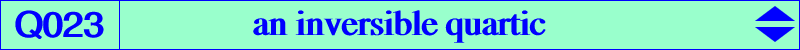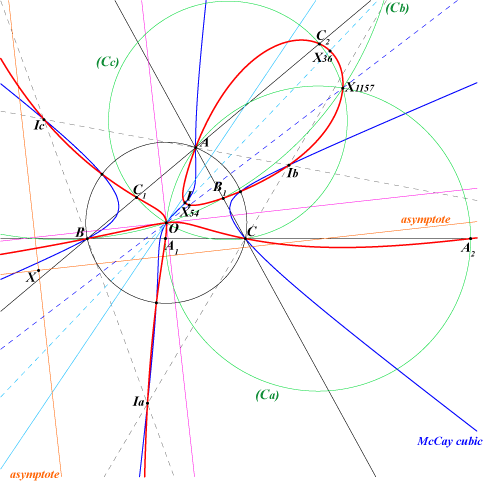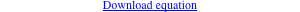too complicated to be written here. Click on the link to download a text file.X(1), X(3), X(36), X(54), X(1157), X(1687), X(1688), X(2574), X(2575) excenters and their inverses in the circumcircle vertices of the circumnormal triangle other points belowQ023 is a circular quartic with singular focus X(2070), the inverse of the nine-point center X(5) in the circumcircle. It has two real asymptotes parallel to those of the Jerabek hyperbola intersecting at the point X = a^4 SA (4SA^2 - b^2c^2) : : not mentioned in the current edition of ETC. X is the barycentric product X3 x X323 and lies on many lines e.g. X2, X567 - X3, X49 - X20, X156 - X30, X110 - X36, X215 - X54, X140 - X125, X539, etc, SEARCH = 4.18620611145902. X is now X(22115) in ETC (2018-09-04). O is a node on Q023 with two tangents parallel to the asymptotes of the Jerabek hyperbola. Q023 meets the Euler line again at two other points E3, E4 that lie on the circum-conic passing through X(54), X(250), X(477), X(1105). The circle with diameter E3, E4 is orthogonal to the circumcircle and its center is X(186). Q023 is invariant in the inversion with respect to the circumcircle. It meets the circumcircle at A, B, C and the vertices U, V, W of the circumnormal triangle, these six points on the McCay cubic. Obviously, the tangents at these six points pass through O. More generally, the polar line with respect to Q023 of any point on the Lemoine cubic K009 passes through O. Q023 contains I, the excenters Ia, Ib, Ic and their inverses X(36), Ja, Jb, Jc. Notice that ABC and JaJbJc are perspective at X(35) not on Q023. Q023 meets the sideline BC at two other points A1, A2 lying on the circle (Ca) passing through A and O, centered at the intersection of BC and the perpendicular bisector of AO. B1, B2 on AC and C1, C2 on AB are defined similarly. Notice that the three circles (Ca), (Cb), (Cc) belong to the same pencil and contain O and X(1157), the inverse of X(54) in the circumcircle. Q023 is the isogonal transform of Q038, an antigonal circular quintic. Q023 is closely related to the Lemoine cubic K009 at least in two ways : a). the third polar curve of O with respect to Q023 is indeed the Lemoine cubic K009. b). if Lo is a line through O meeting again K009 at M and if M' is the inverse of M in the circumcircle, the circle centered at M' orthogonal to the circumcircle meets Lo at two points on Q023 which are naturally inverse in the circumcircle. Moreover, they are harmonically conjugated with respect to O and M. An easy construction of M is the following : the trilinear polar q of any point Q on the line GK envelopes the Kiepert hyperbola and meets the perpendicular bisectors of ABC at Qa, Qb, Qc respectively. The lines AQa, BQb, CQc concur at M on K009. This allows construction of Q023. See the similar inversible quartic Q098. Locus properties : The perpendicular at P to BC meets the line AP* at A'. La is the parallel at A' to AP. Define B', C', Lb, Lc similarly. These lines La, Lb, Lc concur if and only if P lies on the union of the McCay cubic and the orthocubic. Now, if La is the parallel at A' to BC and if Lb, Lc are defined likewise, the lines concur if and only if P lies on the quartic Q023 (Floor van Lamoen and friends, Hyacinthos #5360 & sq.). Q023 is the locus of point Q such that there is a non-isogonal circum-strophoid passing through O. See CL038. Let P be a point and let Oa, Ob, Oc be the reflections of O = X(3) about the lines AP, BP, CP respectively. The triangles ABC and OaObOc are perspective (at Q) if and only if P lies on the circumcircle (in which case Q lies at infinity) or on Q023 (Angel Montesdeoca, private message 2014-12-06). In this latter case, Q lies on Q106. If O is replaced with H, we find Q038 and the line at infinity. Q023 is the locus of P such that the orthocenter H' of the pedal triangle of P lies on the line OP (Angel Montesdeoca, private message 2014-12-13). The locus of H' is a complicated circular septic. See below.A family of related quartics Let X = X(n) be a center on the Euler line of ABC. Let P be a point with circumcevian triangle PaPbPc and denote by Xa, Xb, Xc the center X of triangles PaBC, PbCA, PcAB. When X = X(3), we obviously have Xa = Xb = Xc = X(3) for every P. This is excluded in the sequel. In general, the locus of P such that triangles PaPbPc and XaXbXc are perspective is the circumcircle and a circum-quartic Q(n) passing through X, X(3), X(1) and the excenters, The tangents at A, B, C concur at X. Furthermore, Q(n) passes through A', B', C' defined as follows. If X is the homothetic of G under h(O, T), then A' is the homothetic of A under h(X, T / (T - 3)) which gives OA' = T / (T - 3) HA (vectors). Q(n) meets the sideline B'C' again at A1, A2 on the internal, external bisectors at A of ABC respectively. The points B1, B2 and C1, C2 are defined similarly. Q(n) meets the Euler line at X, X(3) and two other (not always real) points E3, E4 which are inverse in the circumcircle. Hence, the circle with diameter E3, E4 and center the midpoint Ω of E3, E4 is orthogonal to the circumcircle. Special cases : • When X = X(4) hence T = 3, A' is the infinite point of the altitude AH hence Q(4) must split into the line at infinity and a circum-cubic which is the McCay cubic K003. See remark below. • When X tends towards X(3) on the Euler line i.e. T → 0, the limit case is Q(3) = Q023. The table below gives a selection of other remarkable quartics.X = X(n) T Q(n) X(i) on Q(n) for i = Ω Notes X(2) 1 Q026 1, 2, 3, 241, 3229, 5000, 5001 X(468) X(5) 3/2 Q162 1, 3, 4, 5, 186, 523, 847 X(403) 3 asymptotes are parallel to those of K003 X(30) ∞ Q163 1, 3, 30, 40894, 40895 X(10295) 3 asymptotes are parallel to those of K024 X(381) 2 Q164 1, 3, 381, 5627 X(4) 4 asymptotes concurring at X(381) X(25) - Q165 1, 3, 25, 56, 251, 5004, 5005, 40910 X(23) X(24) - 1, 3, 24, 1166 X(2070) X(427) - 1, 3, 427, 5002, 5003 X(858)Remark : when X ≠ X(3) is a point on the Jerabek hyperbola, Q(n) splits into the circumcircle, the line at infinity and a circum-cubic which is pK(X6, X*) where X* is the isogonal conjugate of X hence on the Euler line. These cubics are the Euler cubics of Table 27.